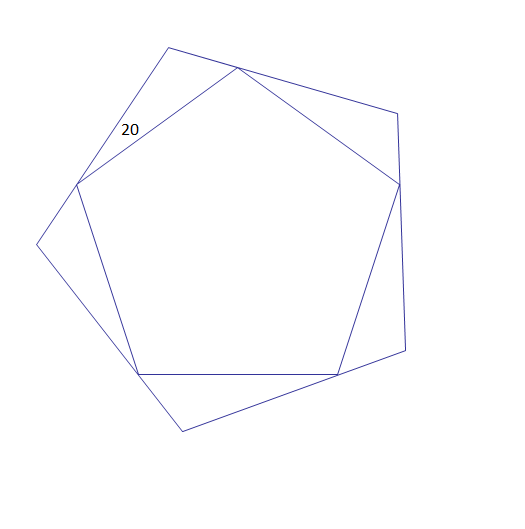# Rotating the PentagonTwo regular pentagons are as shown in the figure. The larger pentagon has been rotated $20^{\circ}$ counter-clockwise with respect to the smaller pentagon, such that all the vertices of the smaller pentagon lie on the sides of the larger pentagon, as shown.

By what percentage is the larger pentagon's side length larger than the side length of the smaller pentagon?

×